# Intro to Crypto 1 - CSCG

Category: Crypto
Difficulty: Baby
Author: black-simon

## Reconnaissance

Files for you to hack along: intro-crypto1.zip

In the description we have a step-by-step guide (original link) (missing some steps) to solve this.

We have a public key and a message.

By reading the guide we know that we have to try to factor the public key.

## Exploitation

At first we have to get the modulus we try to factorize.

To get the modulus, you type:

`openssl rsa -pubin -in pubkey.pem -text -noout`

pubkey.pem being the public key.

You'll get something like this: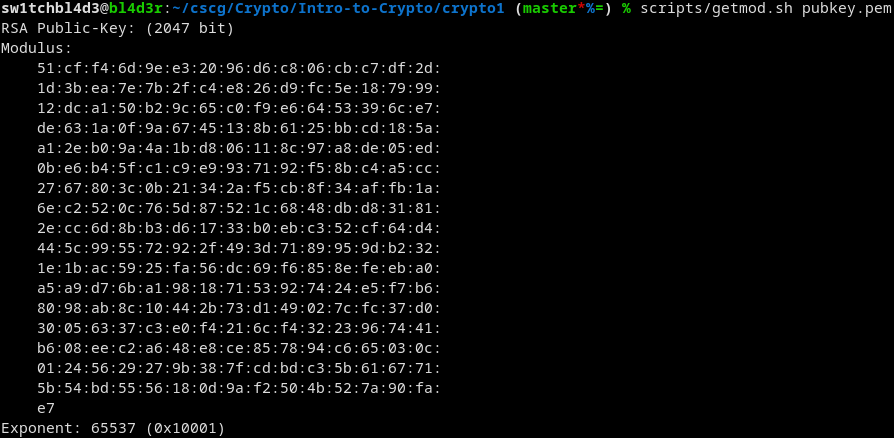(I put the command into a script in scripts/getmod.sh)

Thats not quite the number we need, this is hex and we need a decimal.

I've done that with this small python script:

```
from sys import argv
mod = open(argv, "r")
mod.close()
modin = modin.replace("\n", "").replace(" ", "").replace(":", "")
print(int(modin, 16))
```

Which basically takes a file as a first argument, reads it, removes newlines spaces and colons and converts it.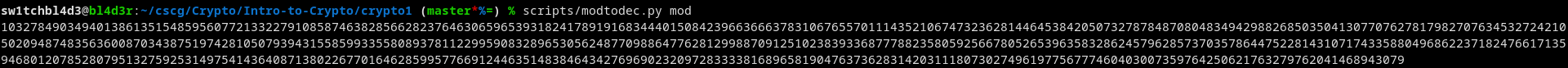You cant really see it because the number is huge (like its supposed to be.)

After that we write a small python script to factor the thing:

```
N = <put n here>

for i in range(2, 1000000):
if N % i == 0:
break
print("p:", i)
print("q:", N//i)
```

This checks if N mod i == 0 (is N divisible by i), and does that for every number from 2 to 1000000.

If that succeeds we have the first prime (and effectively the second one too. (q))

Executing that yields us this: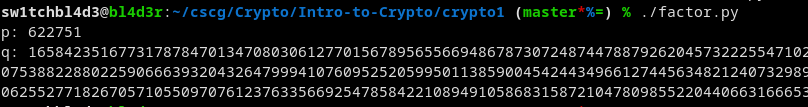(thats not the whole q)

Knowing q and p broke the RSA and we can crack it now.

To do that I stole a few functions from Stack Overflow and made this:

```
p = <insert p>;
q = <insert q>;
e = 65537 # exponent, seen in the getmod.sh
phi = (p-1)*(q-1)

#Took from SO
def egcd(a, b):
if a == 0:
return (b, 0, 1)
g, y, x = egcd(b%a,a)
return (g, x - (b//a) * y, y)

def modinv(a, m):
g, x, y = egcd(a, m)
if g != 1:
raise Exception('No modular inverse')
return x%m

print(modinv(e, phi))
```

This script calculates d, the number we need to decrypt the message. (d = e⁻¹ mod ϕ) ( this website helped a lot with formulas etc....)

Execute that and you'll get d.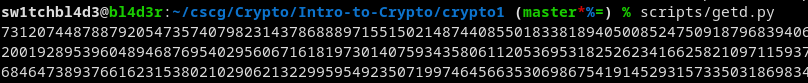Once again not the whole d.

Knowing d will let us decrypt the message as shown in the guide: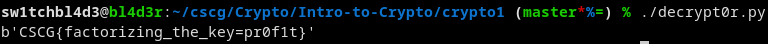And we get this!

## Mitigation

Have both primes be large, not just one, the encryption is as strong as the weakest prime!

~sw1tchbl4d3, 08/04/2020 (dd/mm/yyyy)